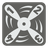[old] Repeating Decimals
Undefined
English

In mathematics, a repeating decimal is a way of representing a rational number. A decimal representation of a number is called a repeating decimal if at some point there is some finite sequence of digits that is repeated infinitely. For example: the decimal representation of 1/3 = 0.3333333… or 0.(3) becomes periodic just after the decimal point, repeating the single-digit sequence "3" infinitely. ....

Input: Two arguments. A numerator and a denominator as integers.

Output: The decimal representation of the fraction in the bracket format as a string.

Example:

```convert(1, 3) == "0.(3)"
convert(5, 3) == "1.(6)"
convert(3, 8) == "0.375"
convert(7, 11) == "0.(63)"
convert(29, 12) == "2.41(6)"
convert(11, 7) == "1.(571428)"
convert(0, 117) == "0."
convert(4, 2) == "2."
```

How it is used: This is the important part for mathematical software. And of you need to help your children with homework.

Precondition:
0 ≤ numerator ≤ 1000
1 ≤ denominator ≤ 1000

You should be an authorized user in order to see the full description and start solving this mission.